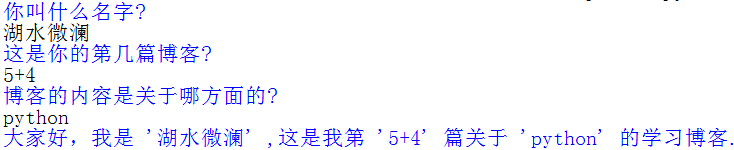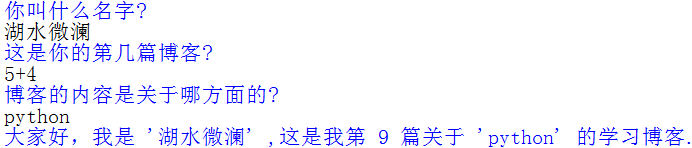• 今天在敲代码的时候发现课本中的“raw_input()”竟然报错了，查阅后发现python3中已经停止了对“raw_input()”函数的使用，之前“raw_input()”的功能合并到了"input()"函数中。 对于还在使用python2的朋友来说，...
今天在敲代码的时候发现课本中的“raw_input()”竟然报错了，查阅后发现python3中已经停止了对“raw_input()”函数的使用，之前“raw_input()”的功能合并到了"input()"函数中。
对于还在使用python2的朋友来说，“raw_input()”和“input()”的主要区别在于：对于输入的数据，前者返回的是string(字符串类型)，后者返回的是数据类型(如：int、float等)。如输入数据【5+3】，对于“raw_input()”，则会输出【‘5+3’】，而对于“input()”,则会输出【‘8’】。
而在python3中："input()"接受任意输入, 将所有输入默认为字符串处理,并返回字符串类型。当输入【5+3】时，不再输出为【‘8’】，而是将输入作为字符串处理，直接输出【‘5+3’】，这点要注意。如果想继续像python2一样直接输出计算结果，应该怎么做呢？下面是简单示例：

print("你叫什么名字?"),
name = input()
print("这是你的第几篇博客?"),
num = input()
print("博客的内容是关于哪方面的?"),
aspect = input()

print("大家好，我是 %r ,这是我第 %r 篇关于 %r 的学习博客."%(name,num,aspect))

输入数据依次为：湖水微澜
5+4
python
运行结果如图：如果想让input()在输入时，像python2一样对数字做计算，而不是输出字符串，可以在输出( print )中使用eval()函数，详见下面的示例：

print("你叫什么名字?"),
name = input()
print("这是你的第几篇博客?"),
num = input()
print("博客的内容是关于哪方面的?"),
aspect = input()

print("大家好，我是 %r ,这是我第 %r 篇关于 %r 的学习博客."%(name,eval(num),aspect))

在代码最后一行末尾，将原来的【num】，改为了【eval(num)】，运行结果如下：PS:上面的代码有些臃肿，实际上简化为如下最佳：

name = input("你叫什么名字?")
num = input("这是你的第几篇博客?")
aspect = input("博客的内容是关于哪方面的?")

print("大家好，我是 %r ,这是我第 %r 篇关于 %r 的学习博客."%(name,eval(num),aspect))


展开全文• 解决办法如下： Python 3 中没有raw_input,可以使用 input
解决办法如下：
Python 3 中没有raw_input,可以使用 input。


展开全文python
• raw_inputinput的区别 版本差异 raw_input——》python2版本 input——》python3版本 输入格式差异 就是raw_input()随便输都是字符串，而input()必须按照Python的规则来~ raw_input() name=raw_input(‘输入...
raw_input与input的区别

版本差异
raw_input——》python2版本
input——》python3版本

输入格式差异
就是raw_input()随便输都是字符串，而input()必须按照Python的规则来~

raw_input()
name=raw_input(‘输入姓名：’)
age=raw_input(‘输入年龄’)
我们输入汉字的姓名和数字的年龄
输入姓名：许嵩
输入年龄：31
许嵩 31
直接输入，变量是str类型
input()
name=input(‘输入姓名：’)
age=input(‘输入年龄：’)
我们还是输入汉字的姓名和数字的年龄
输入姓名：‘许嵩’
输入年龄：31
许嵩 31
姓名为str类型，年龄为int类型，故许嵩加 ’ ’ ，数字直接为数字。
啥时候用raw_input()啥时候用input()?
如果是python2版本，则两种都可以使用
如果是python3版本，则只能使用 input()
建议都使用 input()


展开全文python
• 使用inputraw_input都可以读取控制台的输入，但是inputraw_input在处理数字时是有区别的 纯数字输入 当输入为纯数字时  input返回的是数值类型，如int,float  raw_inpout返回的是字符串类型，string类型 ...
使用input和raw_input都可以读取控制台的输入，但是input和raw_input在处理数字时是有区别的
纯数字输入

当输入为纯数字时

input返回的是数值类型，如int,float
raw_inpout返回的是字符串类型，string类型

输入字符串为表达式

input会计算在字符串中的数字表达式，而raw_input不会。

如输入 “57 + 3”:

input会得到整数60
raw_input会得到字符串”57 + 3”

python input的实现
展开全文python
• Python 版本 2.x 中，raw_input() 会从标准输入（sys.stdin）读取一个输入并返回一个字符串，且尾部的换行符从末尾移除二、区别注意：使用python2.x版本进行相应的练习raw_input()随便输都是字符串，而input()必须...python
• 1、在python2.x中raw_input( )和input( )，两个函数都存在，其中区别为 raw_input( )---将所有输入作为字符串看待，返回字符串类型 input( )-----只能接收“数字”的输入，在对待纯数字输入时具有自己的特性，它...
• ## raw_input和input()

千次阅读 2018-10-17 22:34:10
python 2中有raw_input()，python 3 中已经去掉了raw_input()，将raw_input()和input()整合成input(). raw_input():任何类型都可以接受，将所有的输入都当作字符串类型； input():会根据用户的输入变换相应的类型，...
• inputraw_input区别  1.raw_input的__doc__属性。 raw_input([prompt]) -&gt; string Read a string from standard input. The trailing newline is stripped. If the user hits EOF (Unix: Ctl-D, ...
• 首先明确一点，input()与raw_input()的区别只存在Python2.X环境下,因为在Python3中将Python2中的input()删除了,同时将raw_input()改名为input()。 如果你主要使用Py3，那么你只需关注在使用input（）时所输入的数据...
• 这两个函数均是python的内建函数，都可以读取...例1：>>> raw_input= raw_input("raw_input: ") 2 raw_input: I am raw_input 3 >>> input = input("Input: ") 4 Input: I am input 5 6 Traceback (most recentpython
• ## Python input和raw_input

千次阅读 2017-04-30 15:05:20
使用input()、raw_input()函数  这两个函数在python的内置库里。  input()函数返回一个数值（整型或浮点型）  raw_input()函数返回字符串  用例： value = input('input a int:') print value hello = raw_...python 控制台
• raw_input() 与 input() __ Python 这两个均是 python 的内建函数，通过读取控制台的输入与... 1 >>> raw_input_A = raw_input("raw_input: ") 2 raw_input: abc 3 >>> input_A = input("Input: ") 4 Input: abc
• ## Python3不存在raw_input( )了

万次阅读 多人点赞 2018-07-15 15:50:06
Python3将raw_inputinput进行整合成了input....去除了raw_input()函数....其接受任意输入, 将所有输入默认为字符串处理,并返回字符串类型python3
• 代码中出现： unresolved reference ‘raw_input’ 。 1、问题描述：使用raw_input时，提示 unresolved reference ‘raw_input’ ， 具体如图1所示： 2、问题还原： 之前是好的， 回忆一下， 之前做了什么操作...
• raw_input() 与 input()都是python的内建函数，实现控制台交互式输入，但二者有区别>>> raw_input_A = raw_input("raw_input: ") raw_input: abc >>> input_A = input("Input: ") Input: abcTraceback (most recent...python
• 摘要： raw_input() & input() raw_input的功能是方便的从控制台读入数据。 input与raw_input都是Python的内建函数，实现与用户的交互，但是功能不同。 ...一、raw_input ... raw_input_A = raw...
• ## raw_input()与input()

千次阅读 2019-02-06 16:45:42
python2中，存在raw_input()和input()两个函数 raw_input()将所有输入作为字符串看待，并且返回字符串类型 input()只用于数字的输入，返回所输入数字类型 python3中，只存在input()函数，接收任意类型的输入，...
• 在python2.x中 input():只能输入数字类型（int,float） raw_input()：全部返回字符串 在python3.x中 inputraw_input进行了整合，去除了raw_input，并接受所有输入最作为字符串返回 ...Python
• Python input()和raw_input()的区别 &amp;nbsp; 　区别嘛，就是raw_input()随便输都是字符串，而input()必须按照Python的规则来~ raw_input() name=raw_input('输入姓名：') age=raw_input('...Python内建函数
• python2中有inputraw_input两个方法，而在python3只有一个input方法，作用是在程序中实现用户输入以获取输入结果。 1. python2中，inputraw_input两个方法是有区别的 input()要求用户输入的是合法的Python表达式...python
• 错误截图： name 'raw_input' is not defined python3.x系列没有 raw_input() 函数， raw_input() 是在...python3.x中 input() 和从前的 raw_input() 等效，所以把raw_input()替换为input() 就可以了。 ...解决方案...